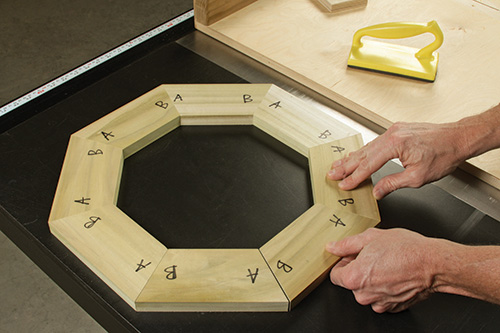# Angles to Cut Octagonal PiecesI’m going to make an octagonal lighthouse and I’m trying to figure out the angle to cut the pieces.

Scott Noyes: The glue-up angle for each piece is 67.5 degrees. The formula for the interior angles of a regular polygon is A (angle) = 180 – (360/n), where n is the number of sides. Hence, the interior angles of an octagon are 135 degrees each. (360 divided by eight is 45. Subtract 45 from 180, and you get 135.) However, each interior angle is split in half to make the two gluing surfaces. For that, you can divide the angle in half to get 67.5 degrees, or use the formula 90 – (180/n) which gives the same answer.

John Brock: To determine the angle, divide the degrees in a circle (360), by the desired number of pieces (8), and then split the angle in two since half will be applied to each side of your stock. For an octagon, the calculated angle is 45 degrees, and 22.5 degrees will be added or subtracted from each side of the stock. Your table saw normally is set at 90 degrees, or perpendicular to the top. Subtract the 22.5 degrees from 90, and you get 67.5 degrees. Set your blade tilt to 67.5.